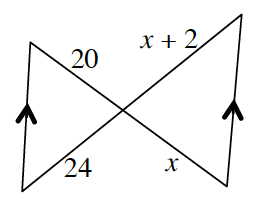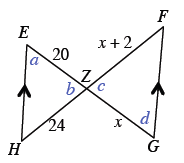### Home > GC > Chapter 6 > Lesson 6.2.3 > Problem6-61

6-61.Examine the triangles in the diagram at right.

1. Are the triangles similar? Justify your conclusion.

There are some parallel lines in the diagram.
Review Math Notes in Lesson 2.1.4 for help identifying the relationships between the angles lettered $a$, $b$, $c$, and $d$ below.2. Solve for $x$. Show all work.

When two triangles are similar, the ratios of corresponding sides are equal.
You could use a proportion.

$x=10$